# Gaussian type orbitals

In quantum chemistry, a Gaussian type orbital (GTO) is an atomic orbital used in linear combinations forming molecular orbitals. GTOs were proposed by Boys as early as 1950, and are at present the basis functions most generally used in quantum chemical program packages.

A GTO is a real-valued function of a 3-dimensional vector r = (x, y, z), the vector pointing from an origin to the position of an electron. Usually the origin is centered on a nucleus in a molecule, but in principle the origin can be anywhere in space. The defining characteristic of Gaussian type orbital is its radial part, which is given by a Gaussian function exp(−αr 2), where r is the length of the electron position vector r and α is a real parameter. The parameter α may be taken from tables of atomic orbital basis sets, which are often contained in quantum chemical computer programs, or can be downloaded from the web. The tables of α may have been prepared by energy minimizations, or by fitting to other (known) orbitals, for instance to Slater type orbitals.

## Angular parts of Gaussian type orbitals

There are two kinds of GTOs in common use: Cartesian and spherical GTOs. They differ in their angular parts.

### Cartesian GTOs

(Unnormalized) Cartesian GTOs are defined by an angular part which is a homogeneous polynomial in the components x, y, and z of the position vector r. That is,$G_{ijk,\alpha}\;\stackrel{\mathrm{def}}{=}\; x^i\,y^j\, z^k\, e^{-\alpha r^2}\quad \hbox{with}\quad i+j+k = n.$

In general there are (n + 1)(n + 2)/2 homogeneous polynomials of degree n in three variables. For instance, for n = 3 we have the following ten Cartesian GTOs,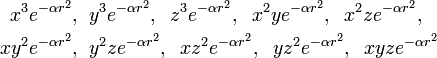\begin{align} x^3 e^{-\alpha r^2},&\;\;y^3 e^{-\alpha r^2},\;\;z^3 e^{-\alpha r^2},\;\;x^2y e^{-\alpha r^2},\;\; x^2z e^{-\alpha r^2},\;\; \\ xy^2 e^{-\alpha r^2},&\;\;y^2z e^{-\alpha r^2},\;\;xz^2 e^{-\alpha r^2},\;\; yz^2 e^{-\alpha r^2},\;\;xyz e^{-\alpha r^2} \end{align}

Note that a set of three p-type (l = 1) atomic orbitals (see hydrogen-like atom for the meaning of p and l ) can be found as linear combinations of nine out of the ten Cartesian GTOs of degree n = 3 (recall that r² = x² + y² + z²):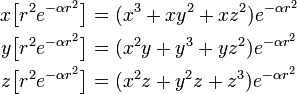\begin{align} x \big[ r^2e^{-\alpha r^2}\big] &= (x^3+xy^2 +xz^2)e^{-\alpha r^2} \\ y \big[ r^2e^{-\alpha r^2}\big] &= (x^2y+y^3 +yz^2)e^{-\alpha r^2} \\ z \big[ r^2e^{-\alpha r^2}\big] &= (x^2z+y^2z +z^3)e^{-\alpha r^2} \\ \end{align}

Observe that the expressions between square brackets only depend on r and hence are spherical-symmetric. The angular parts of these functions are eigenfunctions of the orbital angular momentum operator with quantum number l = 1 [eigenvalue 1(1+1)].

Likewise, a single s-orbital r2exp[−αr2] is "hidden" in a set of six orbitals of degree n = 2:$r^2 e^{-\alpha r^2} \equiv\big(x^2+y^2+z^2\big)e^{-\alpha r^2} \subset e^{-\alpha r^2}\left\{x^2, \, y^2,\,z^2,\,xy,\, xz,\,yz\right\} .$

The general rule is that even powers of r, r2k, can be factored out of the Cartesian GTOs, leaving homogeneous polynomials of order l, which are eigenfunctions of orbital angular momentum with eigenvalue l(l+1). The quantum number l runs as follows$l = \begin{cases} &1, 3, \ldots, n \quad \mathrm{odd}\; n \\ &0, 2, \ldots, n \quad \mathrm{even}\; n \\ \end{cases}$

which are to be multiplied with corresponding factors r2k to obtain the Cartesian GTOs (homogeneous of order n), where$2k = \begin{cases} &n-1, n-3, \ldots, 0 \quad \mathrm{odd}\; n \\ &n, n-2, \ldots, 0 \qquad \mathrm{even}\; n. \\ \end{cases}$

For instance, n = 5 gives l = 1, 3, 5, k = 2, 1, 0, with dimensions: 2(l+1) = 3+7+11 = 21 = (5+1)(5+2)/2. This result has an interesting group theoretical interpretation.

It could be supposed that the "hidden" orbitals with l < n could be an improvement of the AO basis employed in a quantum chemical calculation. However, often they are not. They are prone to give rise to linear dependencies. The spherical GTOs, to be discussed now, are less plagued by the linear dependence problem.

### Spherical GTOs

Cosine- and sine-type regular solid harmonics (normalized to unity) can be defined by the following unitary matrix/vector expression$\begin{pmatrix} ^c\!R_\ell^{|m|} \\ ^s\!R_\ell^{|m|} \end{pmatrix} \equiv \frac{1}{\sqrt{2}} \begin{pmatrix} (-1)^m & \quad 1 \\ -(-1)^m i & \quad i \end{pmatrix} \begin{pmatrix} r^\ell Y_\ell^{|m|} \\ r^\ell Y_\ell^{-|m|} \end{pmatrix}, \qquad m \ne 0,$

and for m = 0: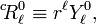$^c\!R_\ell^{0} \equiv r^\ell Y_\ell^{0},$

where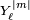${Y_\ell^{|m|} }$ is a spherical harmonic function. For instance the functions for l = 2 are explicitly,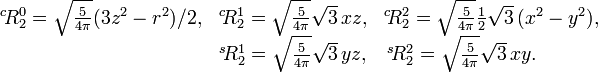$\begin{matrix} ^c\!R_2^0 = \sqrt{\frac{5}{4\pi}}(3z^2-r^2)/2, & ^c\!R_2^1 = \sqrt{\frac{5}{4\pi}}\sqrt{3}\, xz, & ^c\!R_2^2 =\sqrt{\frac{5}{4\pi}}\frac{1}{2}\sqrt{3}\,(x^2-y^2), \\ & ^s\!R_2^1 = \sqrt{\frac{5}{4\pi}}\sqrt{3}\,yz, & ^s\!R_2^2 = \sqrt{\frac{5}{4\pi}}\sqrt{3}\,xy. \qquad\quad \\ \end{matrix}$

See solid harmonics for closed expressions of regular harmonics expressed in Cartesian coordinates. If one expresses regular solid harmonics in spherical polar coordinates, the cosine-type functions contain the factor cos |m| φ and the sine-type functions sin |m| φ. Hence the name. It is of some interest to recall that spherical and solid harmonics span equivalent irreducible representations of the rotation group SO(3).

After this preliminary, we can state that a spherical GTO (radial part unnormalized) is either of cosine-type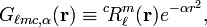$G_{\ell mc, \alpha}(\mathbf{r})\equiv {}^c\!R_\ell^m(\mathbf{r}) e^{-\alpha r^2} ,$

or of sine-type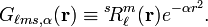$G_{\ell ms, \alpha}(\mathbf{r})\equiv {}^s\!R_\ell^m(\mathbf{r}) e^{-\alpha r^2} .$

It can be proved that the 2l + 1 (orthogonal) spherical GTOs of order l are linear combinations of the Cartesian GTOs of order n = l. That is, the spherical GTOs span a 2l+1 dimensional subspace of the (n+1)(n+2)/2-dimensional Cartesian GTO space spanned by the Cartesian GTOs of order n. In fact, the spherical GTOs span the subspace orthogonal to the subpaces of l < n that—as we have seen above—are subspaces of the Cartesian GTO space. As discussed above, the subspaces with l < n are characterized by the fact that powers of r2 are factored out. For regular solid harmonics a power of r2 cannot be factored out by forming some linear combination [since regular harmonics span an irreducible representation of the group SO(3)].

The quantum chemical methods, for which GTOs are designed, require the calculation of numerous integrals (one- and two-electron integrals, one- through four-center integrals). In practice these integrals are computed on basis of Cartesian GTOs and then transformed to spherical GTOs. This means that basis functions containing powers of r2 are discarded when spherical GTOs are used. Although the CPU cycles required for the integration seem wasted, this discarding shortens the list of integrals. Moreover, spherical GTOs do not become linearly dependent as easily as Cartesian GTOs. As a final advantage we mention that they lead to well-defined selection rules (a priori rules that tell whether certain integrals will vanish), because they are eigenfunctions of orbital angular momentum. This latter property makes results of calculations based on spherical GTOs more transparent and easier to interpret than those based on Cartesian GTOs. It is therefore not surprising that spherical GTOs have been gaining ground on Cartesian GTOs, although some computer packages still give the user a choice.

## Primitive GTOs and contracted sets

The GTOs have two major disadvantages:

1. They do not have a cusp on the nucleus.
2. They fall off too rapidly for large r.

It can be shown that the radial part χ(r) of an AO must have a non-vanishing first derivative (a so-called cusp) at the nucleus: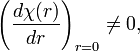$\left(\frac{d \chi(r)}{dr}\right)_{r=0} \ne 0,$

while clearly,$\left(\frac{d \exp(-\alpha r^2)}{dr}\right)_{r=0} = \left(-2\alpha r \exp(-\alpha r^2)\right)_{r=0} = 0.$

It can also be shown that for large r the orbital must fall off exponentially [as exp(−r )]. Slater orbitals (STOs) satisfy both requirements, but are so difficult to integrate that they are hardly used for general molecules. Comparative quantum chemical calculations on diatomic molecules, however, showed that results from GTOs can be as good as those from STOs, provided the GTO basis is much larger (by a factor three to four). GTOs with large α are very much concentrated around the origin (in the limit of infinite α they tend to a Dirac delta function) and can mimick the correct cusp. GTOs with small α are very diffuse (spread out) and can describe the behavior of a molecular orbital (MO) at large r.

Large basis sets lead to large integral lists (if m is the total number of GTOs, the length of the list is proportional to m4) and costly further calculations. For example, a post-Hartree-Fock method like CCSD(T) requires computer time proportional to m7. It turns out that a reliable description of atomic inner shell orbitals requires a fair amount of basis functions, but it also turns out that these orbitals are described by linear combinations of GTOs that hardly change from molecule to molecule. Further it appears—not surprisingy—that the form of the inner shell orbitals do not affect chemical binding very much. These considerations led to the introduction of contracted sets of primitive GTOs.

A contracted set is a predetermined linear combination of radial parts of GTOs (that are on the same center). Indexing different parameters α by s, the definition of a contracted set χ(r) is,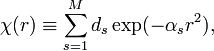$\chi(r) \equiv \sum_{s=1}^M d_s \exp(-\alpha_s r^2),$

where M is the length of the contraction. The contraction coefficients are ds. The functions${\exp(-\alpha_s r^2) }$ are called primitive GTOs. The contraction coefficients ds and the corresponding exponents αs are tabulated side-by-side and together they define the (contracted) GTO χ(r). In a quantum chemical calculation it is χ(r) that is considered to be the radial part of an AO. It can be multiplied by a homogeneous polynomial in x, y, and z to become a Cartesian GTO, or χ(r) can be multiplied by a regular solid harmonic to become a spherical GTO. The number of contracted sets on a single nucleus that can be included in a calculation is in principle unlimited. In practice, computer time and linear dependence form bounds to the number.

In the early Gaussian-based computer programs the contractions were usually segmented, that is to say, a primitive function appeared in one contraction only. Nowadays general contractions are more common, a primitive GTO may appear in more than one contraction.

## Gaussian product rule

The main reason that Gaussians are easy to handle is the Gaussian product rule. In almost all quantum chemical integrals one meets two center distributions, that is, product functions of the form$\Phi(r) \equiv \exp(-\alpha r_A^2) \exp(-\alpha r_B^2)$

with$r_A = |\mathbf{r} - \mathbf{A}|,\quad\hbox{and}\quad r_B = |\mathbf{r} - \mathbf{B}|$

where A and B are position vectors of the different fixed points A and B in the molecule (usually the positions of nuclei). The vector r is the position vector of an electron.

The Gaussian product rule states that Φ(r) is proportional to a single Gauss function of r centered somewhere on the line A—B. More precisely,$\Phi(r) \equiv e^{-\alpha r_A^2}\; e^{-\beta r_B^2} = e^{- \frac{\alpha\beta}{\alpha + \beta} (B-A)^2 }\; e^{-(\alpha+\beta)r_C^2}$

with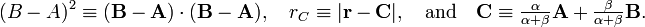$(B-A)^2 \equiv (\mathbf{B}-\mathbf{A})\cdot(\mathbf{B}-\mathbf{A}),\quad r_C \equiv |\mathbf{r}-\mathbf{C}|,\quad\hbox{and}\quad \mathbf{C} \equiv \tfrac{\alpha}{\alpha+\beta} \mathbf{A} +\tfrac{\beta}{\alpha+\beta} \mathbf{B}.$

Note that the position of C is given by the following parametric equation for the line segment between A and B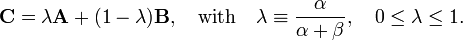$\mathbf{C} = \lambda \mathbf{A} + (1-\lambda) \mathbf{B},\quad \hbox{with}\quad \lambda \equiv \frac{\alpha}{\alpha+\beta}, \quad 0\le \lambda \le 1.$

The angular parts of a two-center distribution can be expressed relatively simply with respect to the center C, so that the rule simplifies an integral over a two-center distribution to a one-center integral. An analogous result holds for a four-center integral (which depends on two-center distributions of two different electrons). A four-center integral is reduced to a two-center integral by application of the Gaussian product rule.

The proof of the rule is simple. Considering the exponents only, we write$\alpha (\mathbf{r}-\mathbf{A}) \cdot (\mathbf{r}-\mathbf{A}) + \beta (\mathbf{r}-\mathbf{B}) \cdot (\mathbf{r}-\mathbf{B}) = P + \gamma (\mathbf{r}-\mathbf{C}) \cdot (\mathbf{r}-\mathbf{C})$

and determine P, γ and C by expanding and equating coefficients of r. Terms in r2:$\alpha r^2 + \beta r^2 = \gamma r^2 \Longrightarrow \gamma = \alpha + \beta.$

Terms linear in r :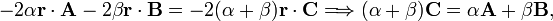$-2\alpha \mathbf{r}\cdot\mathbf{A} -2\beta \mathbf{r}\cdot\mathbf{B} = -2(\alpha+\beta) \mathbf{r}\cdot\mathbf{C} \Longrightarrow (\alpha+\beta)\mathbf{C} = \alpha\mathbf{A}+\beta\mathbf{B},$

which gives the result to be proved for C. Finally equating the constant terms: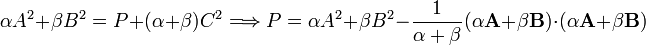$\alpha A^2 + \beta B^2 = P + (\alpha+\beta) C^2 \Longrightarrow P = \alpha A^2 + \beta B^2 - \frac{1}{\alpha + \beta}(\alpha \mathbf{A} + \beta\mathbf{B})\cdot(\alpha \mathbf{A} + \beta\mathbf{B})$

Hence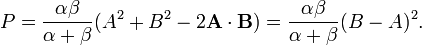$P = \frac{\alpha\beta}{\alpha + \beta}(A^2 + B^2 -2 \mathbf{A}\cdot \mathbf{B}) = \frac{\alpha\beta}{\alpha + \beta} (B-A)^2.$

Putting these findings as exponents in the exponential functions gives the result to be proved.